## Finding Centroid point from shape file and labelling map

Finding Centroid point from shape file and labelling map

# Finding Centroid point from shape file and map data labelling

In this test I try to find centroid point in shp file. In additionally I will show how to use this centroid point information when to labelling area name into map.
R Library PBSmapping offers wide range of functions to do that job.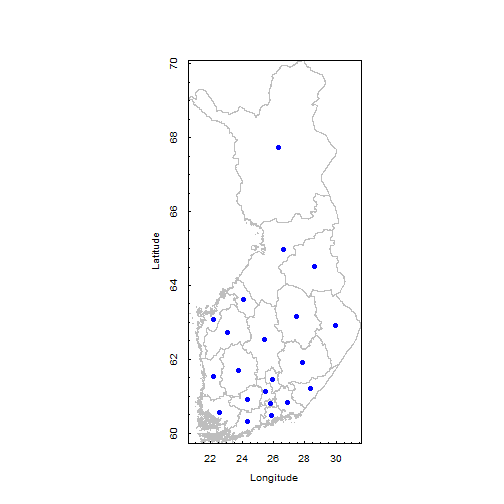## Needed Library

``````require(rgdal)
``````
``````## Loading required package: rgdal
``````
``````## Loading required package: sp
``````
``````## Warning: package 'sp' was built under R version 2.15.1
``````
``````## Geospatial Data Abstraction Library extensions to R successfully loaded
## Loaded GDAL runtime: GDAL 1.9.0, released 2011/12/29 Path to GDAL shared
## files: C:/Program Files/R/R-2.14.2/library/rgdal/gdal Loaded PROJ.4
## runtime: Rel. 4.7.1, 23 September 2009, [PJ_VERSION: 470] Path to PROJ.4
## shared files: C:/Program Files/R/R-2.14.2/library/rgdal/proj
``````
``````require(PBSmapping)
``````
``````## Loading required package: PBSmapping
``````
``````## ----------------------------------------------------------- PBS Mapping
##
## PBS Mapping comes with ABSOLUTELY NO WARRANTY; for details see the file
## COPYING. This is free software, and you are welcome to redistribute it
## under certain conditions, as outlined in the above file.
##
## A complete user guide 'PBSmapping-UG.pdf' is located at C:/Program
## Files/R/R-2.14.2/library/PBSmapping/doc/PBSmapping-UG.pdf
##
## To see demos, type '.PBSfigs()'.
##
## Packaged on 2012-03-01 Pacific Biological Station, Nanaimo
## -----------------------------------------------------------
``````
``````require(maptools)
``````
``````## Loading required package: maptools
``````
``````## Loading required package: foreign
``````
``````## Loading required package: lattice
``````
``````## Checking rgeos availability: TRUE
``````

## Importing Shapefile

``````FIN_adm2.pb <- importShapefile("FIN_adm2")
``````

ImportShapefile reads the .prj file if it exists, but it
does not adopt the proj4 format

``````proj.abbr <- attr(FIN_adm2.pb, "projection")
# abbreviated projection info
``````

print(proj.abbr)

## Generate map using PBSmapping plotting functions

``````plotPolys(FIN_adm2.pb, projection = proj.abbr,
border = "gray", xlab = "Longitude", ylab = "Latitude")
``````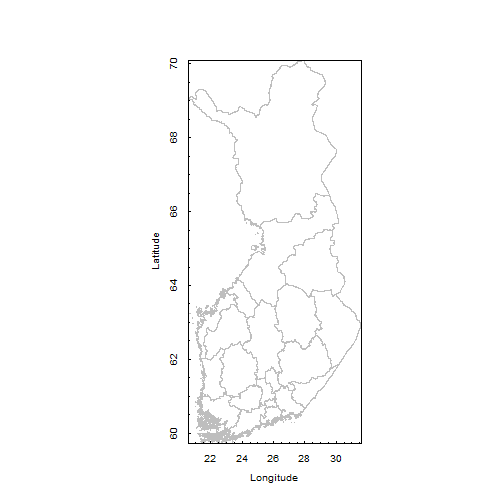## Calculate the centroids of adm2 area

``````FIN.centroids2 <- calcCentroid(FIN_adm2.pb, rollup = 1)
``````

Check results

``````str(FIN.centroids2)
``````
``````## Classes 'PolyData' and 'data.frame': 21 obs. of  3 variables:
##  \$ PID: int  1 2 3 4 5 6 7 8 9 10 ...
##  \$ X  : num  29.9 27.5 26 27.9 26.3 ...
##  \$ Y  : num  62.9 63.2 61.4 61.9 67.7 ...
##  - attr(*, "projection")= chr "LL"
``````

## Plot the centroids against the source polygons

In this purpose we use the PBSMapping package graphics routines. If you want you can plot these centroid point in to the map

``````plotPolys(FIN_adm2.pb, projection = proj.abbr,
border = "gray", xlab = "Longitude", ylab = "Latitude")
addPoints(FIN.centroids2, col = "blue", pch = 19)
``````check the variables from imported shape file

``````str(FIN_adm2.pb)
``````
``````## Classes 'PolySet' and 'data.frame':  136271 obs. of  5 variables:
##  \$ PID: int  1 1 1 1 1 1 1 1 1 1 ...
##  \$ SID: int  1 1 1 1 1 1 1 1 1 1 ...
##  \$ POS: int  1 2 3 4 5 6 7 8 9 10 ...
##  \$ X  : num  28.5 28.5 28.5 28.5 28.6 ...
##  \$ Y  : num  63.9 63.9 63.9 63.9 63.9 ...
##  - attr(*, "PolyData")=Classes 'PolyData' and 'data.frame':  21 obs. of  12 variables:
##   ..\$ PID      : int  1 2 3 4 5 6 7 8 9 10 ...
##   ..\$ ID_0     : int  78 78 78 78 78 78 78 78 78 78 ...
##   ..\$ ISO      : Factor w/ 1 level "FIN": 1 1 1 1 1 1 1 1 1 1 ...
##   ..\$ NAME_0   : Factor w/ 1 level "Finland": 1 1 1 1 1 1 1 1 1 1 ...
##   ..\$ ID_1     : int  1 1 1 1 2 3 3 4 4 4 ...
##   ..\$ NAME_1   : Factor w/ 5 levels "Eastern Finland",..: 1 1 1 1 2 3 3 4 4 4 ...
##   ..\$ ID_2     : int  1 2 3 4 5 6 7 8 9 10 ...
##   ..\$ NAME_2   : Factor w/ 19 levels "Central Finland",..: 8 9 13 17 7 5 10 3 6 13 ...
##   ..\$ NL_NAME_2: Factor w/ 0 levels: NA NA NA NA NA NA NA NA NA NA ...
##   ..\$ VARNAME_2: Factor w/ 19 levels "Etelä-Karjala|Södra Karelen",..: 12 14 15 3 10 5 13 4 9 15 ...
##   ..\$ TYPE_2   : Factor w/ 1 level "Maakunta|landskap": 1 1 1 1 1 1 1 1 1 1 ...
##   ..\$ ENGTYPE_2: Factor w/ 1 level "Region": 1 1 1 1 1 1 1 1 1 1 ...
##  - attr(*, "parent.child")= num  1 1 1 1 0 1 0 0 0 0 ...
##  - attr(*, "shpType")= int 5
##  - attr(*, "prj")= __truncated__
##  - attr(*, "projection")= chr "LL"
``````

To read dbf file we need foreign library

``````library(foreign)
``````

reading shape file data from dbf file (ESRI files include normally: shp, dbf, prj, csv and shx data files)

``````labelData <- read.dbf("FIN_adm2.dbf", as.is = TRUE)
``````

Data checking

``````head(labelData)
``````
``````##   ID_0 ISO  NAME_0 ID_1          NAME_1 ID_2            NAME_2 NL_NAME_2
## 1   78 FIN Finland    1 Eastern Finland    1     North Karelia      <NA>
## 2   78 FIN Finland    1 Eastern Finland    2     North Savonia      <NA>
## 3   78 FIN Finland    1 Eastern Finland    3 Päijänne Tavastia      <NA>
## 4   78 FIN Finland    1 Eastern Finland    4  Southern Savonia      <NA>
## 5   78 FIN Finland    2         Lapland    5           Lapland      <NA>
## 6   78 FIN Finland    3            Oulu    6            Kainuu      <NA>
##                         VARNAME_2            TYPE_2 ENGTYPE_2
## 1   Pohjois-Karjala|Norra Karelen Maakunta|landskap    Region
## 2      Pohjois-Savo|Norra Savolax Maakunta|landskap    Region
## 3 Päijät-Häme|Päijänne Tavastland Maakunta|landskap    Region
## 4        Etelä-Savo|Södra Savolax Maakunta|landskap    Region
## 5                  Lappi|Lappland Maakunta|landskap    Region
## 6               Kainuu|Kajanaland Maakunta|landskap    Region
``````

merging shape files area name and centroid points (x and y coordinates) labelData as data frame

``````labelData <- as.data.frame(labelData)
``````

merging labelData and centroid data table fields

``````label <- data.frame(labelData\$ID_2, FIN.centroids2\$X,
FIN.centroids2\$Y, labelData\$NAME_2)
``````

Event data function needs right column names

``````names(label) <- c("EID", "X", "Y", "label")
``````

Note: Above routine used in eventdata function

#######names(label)<- c(“PID”, “X”, “Y”, “label”) –> USED IN POLYDATA FUNCTION

do the right data format into labelling

``````data <- as.EventData(label, projection = NULL,
zone = NULL)
``````

## Labelling into map

#col = 1 –> font color 1=black, 2=….
#font = 3 –> font size
#cex = 0.5 –>expansion factor

``````plotPolys(FIN_adm2.pb, projection = proj.abbr,
border = "gray", xlab = "Longitude", ylab = "Latitude")

col = 1, font = 3, cex = 0.5)
``````
``````## Warning: The data's 'projection' attribute (NULL) differs from the
## projection of the plot region (LL).
``````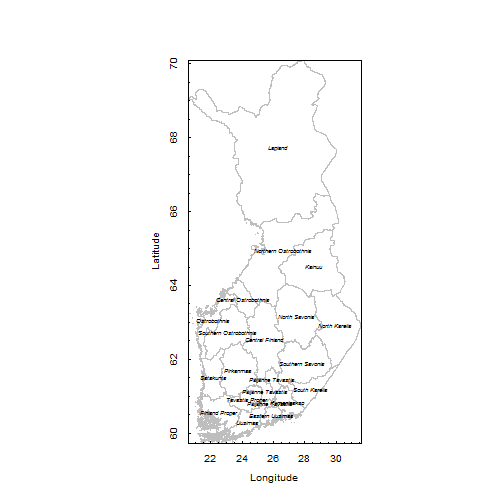Have fun with mapping and RStudio

Marko

ps. This post is produced by using RStudio and knitr. Thanks to Yihui Xie for amazing work with this issue.

# R and Eurostat bulk data

In this Exercise I am testing Eurostat bulk data source and plot these data into Heatmap. Let’s try with this data:
“Harmonised unemployment rates (%) – monthly data (ei_lmhr_m)”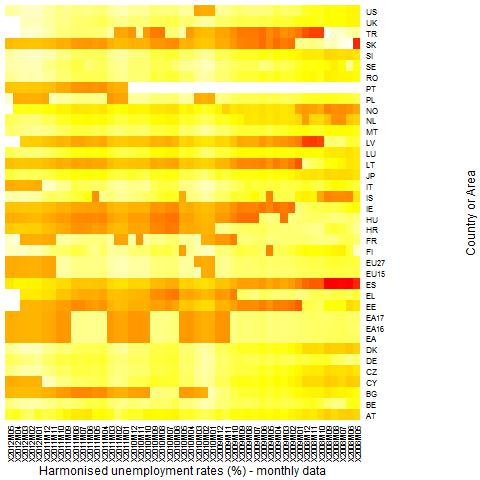#You will also find Eurostat Data source from here:

``` #I will automate this data downloading and extracting, but just now this is semiautomatic # create download directory and set it .exdir = 'c:/data/tmp2' # put there your own data folder dir.create(.exdir) .file = file.path(.exdir, 'ei_lmhr_m.tsv.gz') # change this `````` # download file url = 'http://epp.eurostat.ec.europa.eu/NavTree_prod/everybody/BulkDownloadListing?sort=1&downfile=data `````` %2Fei_lmhr_m.tsv.gz' download.file(url, .file) `````` # untar it (Note: I do not know why I got error message: Error in getOct(block, 100, 8) : invalid octal digit) untar(.file, compressed = 'gzip', exdir = path.expand(.exdir)) `````` # Argh...something going wrong with this step, so I have to manipulate just downloaded data. First I remove comma `````` # from very first variables and etc... I always use Notetab light as a Text editor in this kind of task. `````` # Reading file into R. Please refer here your own data folder... input <- read.table("c:/data/tmp2/ei_lmhr_m.tsv", header=TRUE, sep="\t", na.strings=":", dec=".", strip.white=TRUE) `````` #just checking head(input) `````` `````` # LM-UN-T-TOT = Unemployment rate according to ILO definition - Total rate`````` # NSA = not seasonally adjusted input<- input[which(input\$indic=="LM-UN-T-TOT"),] input<- input[which(input\$s_adj=="NSA"),] `````` #giving appropriate names in to the heatmap (without this manouver there will be only row id) row.names(input) <- input\$geo.time `````` #just checking head(input) `````` #Column selection. We will get data between time period 05/2008 - 05/2012 `````` input2 <- input[,5:53] `````` # data frame must change into data matrix  to produce heatmap. input_matrix <- data.matrix(input2) `````` #heatmap is almost here input_heatmap <- heatmap(input_matrix, Rowv=NA, Colv=NA, col = heat.colors(256), scale="column", margins=c `````` (5,10), xlab = "Harmonised unemployment rates (%) - monthly data", ylab= "Country or Area") `````` `````` #saving heatmap into folder jpeg("G:/data/home/2012/marko/blogi_rbloggerqvist/data/eurostat/Harmonised unemployment rates percent `````` monthly data.jpg") input_heatmap <- heatmap(input_matrix, Rowv=NA, Colv=NA, col = heat.colors(256), scale="column", margins=c `````` (5,10), xlab = "Harmonised unemployment rates (%) - monthly data", ylab= "Country or Area") dev.off() `````` Have fun, Marko```

## R and population maps in Finland 31.5.2012

This example source is almost same like this. I used at first time sorvi-package. In this example I modified little bit original and try to compare MML and GADM shape – files. As we know there is some need to updates into GADM shapefiles (as you will see). Datafiles source date is 31.05.2012 from Väestörekisterikeskus. Thanks to Louhos -people and their invaluable work within open -data project.

# library sorvi You will find there

library(sorvi)
library(rgeos)
library(rgdal)
if (!gpclibPermit()) { gpclibPermit() }

# Finland map and municipility data at gadm-format

##now we use also MML as data source (shape) because of compare shape files
# (C) MML 2011
data(MML)
sp <- MML[[“1_milj_Shape_etrs_shape”]][[“kunta1_p”]]

# vaestorekisteri population data from data source vrk.fi  31.05.2012
vrek <- GetPopulationRegister(“http://vrk.fi/default.aspx?docid=6706&site=3&id=0”)

# Attach vrk data into map object and
# set population as zero where na

sp\$asukkaita <- log10(rowSums(vrek[sp\$Kunta, c(“Miehet”, “Naiset”)]))
sp\$asukkaita[is.na(sp\$asukkaita)] <- 0

# male and female share

sp\$miehet.osuus <- vrek[sp\$Kunta, “Miehet”]/vrek[sp\$Kunta, “Yhteensa”]
sp\$naiset.osuus <- vrek[sp\$Kunta, “Naiset”]/vrek[sp\$Kunta, “Yhteensa”]

sp_summary_male <- summary(vrek[sp\$Kunta, “Miehet”]/vrek[sp\$Kunta, “Yhteensa”])
sp_summary_female <- summary(vrek[sp\$Kunta, “Naiset”]/vrek[sp\$Kunta, “Yhteensa”])

#some summary stat

sp_summary_male
sp_summary_female

hist_sp_male <- hist(sp\$miehet.osuus)
hist_sp_female <- hist(sp\$naiset.osuus)

plot(density(sp\$miehet.osuus,na.rm=TRUE))
plot(density(sp\$naiset.osuus,na.rm=TRUE))

#saving hist figure into file …\r_maps
jpeg(“…/hist.jpg”)
layout(matrix(1:4,2,2))
hist_sp_male <- hist(sp\$miehet.osuus)
hist_sp_female <- hist(sp\$naiset.osuus)
dev.off()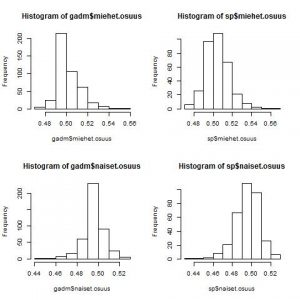#saving density figre into file …\r_maps (replace … as your fav. folder)
jpeg(“…/density.jpg”)
layout(matrix(1:4,2,2))
plot(density(sp\$miehet.osuus,na.rm=TRUE))
plot(density(sp\$naiset.osuus,na.rm=TRUE))
dev.off()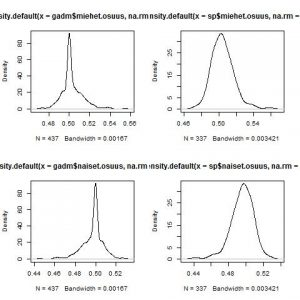# set share  50% male/female
# where na

#same with MML

sp\$miehet.osuus[is.na(sp\$miehet.osuus)] <- 0.5
sp\$naiset.osuus[is.na(sp\$naiset.osuus)] <- 0.5

varname1 <- “naiset.osuus”
at1 <- seq(0.5 – interval1, 0.5 + interval1, length = 100)

#border of interval MML

varname2 <- “naiset.osuus”
interval2 <- max(abs(sp[[varname2]] – 0.5))
at2 <- seq(0.5 – interval2, 0.5 + interval2, length = 100)

#PLOTTING INTO FILE

q1 <- PlotShape(gadm, varname1, type = “twoway”, at = at1, main = “Share of women (red) and men (blue) 31.05.2012 n=437 (GADM)”)
dev.off()

jpeg(“j:/todo/r_maps/mml_280612.jpg”)
q2 <- PlotShape(sp, varname2, type = “twoway”, at = at2, main = “Share of women (red) and men (blue) 31.05.2012 n=337 (MML)”)
dev.off()

#Which one is best?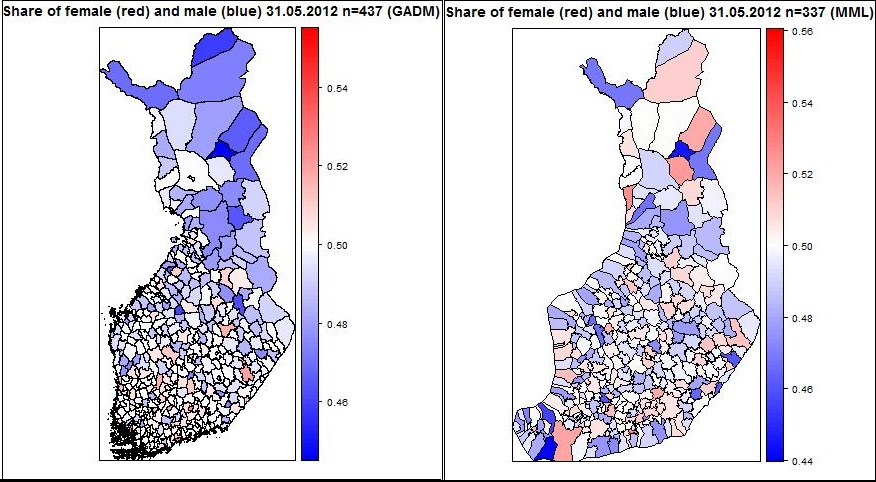GADM projection is not like “normal” and MML coastline is little bit odd, but I am patiently looking forward to improvement  into this issue….

ME/290612

# Using R as visualizing Volatility Index VIX

On September 22, 2003, the CBOE began disseminating price level information using revised methodology for the CBOE Volatility Index, VIX. A spreadsheet with more than 13 years of price history data using this new methodology is now available.

## VIX?

In 1993, the Chicago Board Options Exchange® (CBOE®) introduced the CBOE Volatility Index®, VIX®, and it quickly became the benchmark for stock market volatility. It is widely followed and has been cited in hundreds of news articles in the Wall Street Journal, Barron’s and other leading financial publications. Since volatility often signifies financial turmoil, VIX is often referred to as the “investor fear gauge”.
Source: http://www.cboe.com/micro/vix/faq.aspx#1

So, I found this example from there. I change it little bit. In this exampele it use whole time series you will found there. Now let’s look at closer this amazing approach…
``` #required library require(quantmod) require(ggplot2) require(reshape2) require(plyr) require(scales) `````` # download data source input <- read.table("http://www.cboe.com/publish/ScheduledTask/MktData/datahouse/vixcurrent.csv", header=TRUE, sep=",", na.strings="NA", dec=".", strip.white=TRUE) `````` # creating dataframe dat<-data.frame(date=index(VIX),VIX) #look what we get head(dat) `````` #date conversation input\$date <- as.Date(input\$Date, "%m/%d/%Y") `````` ## below some example do to that ## read in date/time info in format 'm/d/y h:m:s' ## dates <- c("02/27/92", "02/27/92", "01/14/92", "02/28/92", "02/01/92") ## times <- c("23:03:20", "22:29:56", "01:03:30", "18:21:03", "16:56:26") ## x <- paste(dates) ## y <- strptime(x, "%m/%d/%y") `````` # extract year input\$year<-as.numeric(as.POSIXlt(input\$date)\$year+1900) `````` # and  the month too OK input\$month<-as.numeric(as.POSIXlt(input\$date)\$mon+1) `````` # monts into right order and giving factor name input\$monthf<-factor(input\$month,levels=as.character(1:12),labels=c("Jan","Feb","Mar","Apr","May","Jun","Jul","Aug","Sep","Oct","Nov","Dec"),ordered=TRUE) `````` # week days input\$weekday = as.POSIXlt(input\$date)\$wday `````` # week day ordering and gibing factor name (weekdays) input\$weekdayf<-factor(input\$weekday,levels=rev(1:7),labels=rev(c("Mon","Tue","Wed","Thu","Fri","Sat","Sun")),ordered=TRUE) `````` # yearmonth input\$yearmonth<-as.yearmon(input\$date) input\$yearmonthf<-factor(input\$yearmonth) `````` # week of year by rows input\$week <- as.numeric(format(input\$date,"%W")) `````` # making week to start at number 1 input<-ddply(input,.(yearmonthf),transform,monthweek=1+week-min(week)) `````` # Ok, Now we are ready to plot `````` P<- ggplot(input, aes(monthweek, weekdayf, fill = VIX.Close)) + geom_tile(colour = "white") + facet_grid(year~monthf) + scale_fill_gradient(low="yellow", high="red") + opts(title = "Heatmap - view of future expected stock market volatility (risk)") + xlab("Week of Month") + ylab("") `````` #show the plot P `````` # save the picture # example - output graph to jpeg file jpeg("heatmaps.jpg") P dev.off() `````` ...and output will look like this:```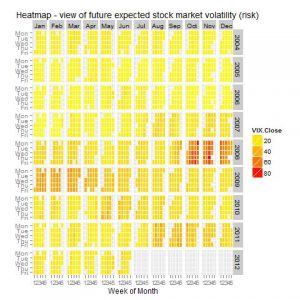That’s it,

Cheers, Marko

# Earth quakes of the last 30 days

These example come from here. I follow instruction how to plot earthquage data into world map between 24.5. – 23.6.2012. My first test is not succesfull, so I make my copy paste tricks. Final result show now “right” magnitude.```## Plot world wide earth quakes of the last 30 days with magnitude >= 4.0 library(googleVis) library(XML) ## Get earthquake data of the last 30 days ## That is ok, put for some reason I do not get right magnitude into picture. eq=readHTMLTable(readLines("http://www.iris.edu/seismon/last30.html")) eq <- eq[] ## extract the eq table summary(eq) #something wrong with this  last30 file #I have to copy and paste web-page data into Notepad and saved this into file earthquake.txt #eq <- read.table("j:/todo/R_geodata/earthquake.txt", header=TRUE, sep="\t", na.strings="NA", dec=".", strip.white=TRUE) #WEB-server datafile note I change MAG into magni eq <- read.table("http://ekqvist.goeuropeinfo.com/rbloggerqvist/data/earthq/earthquake.txt", header=TRUE, sep="\t", na.strings="NA", dec=".", strip.white=TRUE)```

```# lat and lon must be the same variable eq\$loc=paste(eq\$LAT, eq\$LON, sep=":") ## create a lat:long location variable```

```# making geomap earthq <- gvisGeoMap(eq, locationvar="loc", numvar="magni", hovervar="DATE", options=list(dataMode="markers"))```

```#plotting results into html file #plot(earthq) cat(earthq\$html\$chart, file="j:/todo/R_geodata/earthq.html")```

`By clicking this link you will see the result file.`

Greetings,

Marko

# Helppoa kuin heinänteko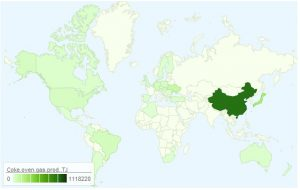Here in Finland we have phrase: ” Helppoa kuin heinänteko”. Now my purpose is to indicate what is the relation between this phrase and using R library googleVis.
In this example we use UN datasets from this source.

All datasets used in this examples You could download bloggerqvist server. Of course I encourage You to find appropriate UN dataset and find data within your interest.

## Example1: Coke Oven gas production 2006-2009 (TJ)

```################################################################ UN data source: http://data.un.org/ Coke oven gas - production ################################################################ # setting amazing library library(googleVis) ################################################################ # download data input <- read.table("http://energy.goeuropeinfo.com/data/un_data/UNdata_Export_20120622_210218958.csv", header=TRUE, sep=";", na.strings="NA", dec=",", strip.white=TRUE)```

```#selecting yearly data select09<- input[which(input\$Year=="2009"),] select08<- input[which(input\$Year=="2008"),] select07<- input[which(input\$Year=="2007"),] select06<- input[which(input\$Year=="2006"),] `````` #selecting variables Map09<- data.frame(select09\$Country.or.Area, select09\$Quantity) Map08<- data.frame(select08\$Country.or.Area, select08\$Quantity) Map07<- data.frame(select07\$Country.or.Area, select07\$Quantity) Map06<- data.frame(select06\$Country.or.Area, select06\$Quantity) `````` #change variable name names(Map09)<- c("Country", "Coke-oven gas prod. TJ") names(Map08)<- c("Country", "Coke-oven gas prod. TJ") names(Map07)<- c("Country", "Coke-oven gas prod. TJ") names(Map06)<- c("Country", "Coke-oven gas prod. TJ") `````` #year 2009 unmap09=gvisGeoMap(Map09, locationvar="Country", numvar="Coke-oven gas prod. TJ", options=list(height=350, dataMode='regions', chartid="Coke-oven prod 2009")) #year 2008 unmap08=gvisGeoMap(Map08, locationvar="Country", numvar="Coke-oven gas prod. TJ", options=list(height=350, dataMode='regions')) #year 2007 unmap07=gvisGeoMap(Map07, locationvar="Country", numvar="Coke-oven gas prod. TJ", options=list(height=350, dataMode='regions')) #year 2006 unmap06=gvisGeoMap(Map06, locationvar="Country", numvar="Coke-oven gas prod. TJ", options=list(height=350, dataMode='regions')) `````` #testing that all is ok plot(unmap09) plot(unmap08) plot(unmap07) plot(unmap06) `````` # saving just created map into html-file cat(unmap09\$html\$chart, file="j:/todo/UN/undata_cokeoven_prod_TJ_2009.html") cat(unmap08\$html\$chart, file="j:/todo/UN/undata_cokeoven_prod_TJ_2008.html") cat(unmap07\$html\$chart, file="j:/todo/UN/undata_cokeoven_prod_TJ_2007.html") cat(unmap06\$html\$chart, file="j:/todo/UN/undata_cokeoven_prod_TJ_2006.html")```

Results file looks like this:
Map 2009, 2008, 2007 and 2006

Have fun,
Marko

…..oops Here Is also an another example….

## Example2: Energy use (kg oil eqv per capita)```################################################################ UN data source: http://data.un.org/ energy use ################################################################ library(googleVis) input <- read.table("http://energy.goeuropeinfo.com/data/UNdata_Export_20120623_070334184_energyuse_kg_eqv_oil_per_capita.txt", header=TRUE, sep=";", na.strings="NA", dec=",", strip.white=TRUE) # select year into matrices select09<- input[which(input\$Year=="2009"),] select08<- input[which(input\$Year=="2008"),] select07<- input[which(input\$Year=="2007"),] select06<- input[which(input\$Year=="2006"),] `````` #select area and value field Map09<- data.frame(select09\$Country.or.Area, select09\$Value) Map08<- data.frame(select08\$Country.or.Area, select08\$Value) Map07<- data.frame(select07\$Country.or.Area, select07\$Value) Map06<- data.frame(select06\$Country.or.Area, select06\$Value) `````` names(Map09)<- c("Country", "Energy Use Eqv oil kg per capita") names(Map08)<- c("Country", "Energy Use Eqv oil kg per capita") names(Map07)<- c("Country", "Energy Use Eqv oil kg per capita") names(Map06)<- c("Country", "Energy Use Eqv oil kg per capita") `````` unmap09=gvisGeoMap(Map09, locationvar="Country", numvar="Energy Use Eqv oil kg per capita", options=list(height=350, dataMode='regions')) unmap08=gvisGeoMap(Map08, locationvar="Country", numvar="Energy Use Eqv oil kg per capita", options=list(height=350, dataMode='regions')) unmap07=gvisGeoMap(Map07, locationvar="Country", numvar="Energy Use Eqv oil kg per capita", options=list(height=350, dataMode='regions')) unmap06=gvisGeoMap(Map06, locationvar="Country", numvar="Energy Use Eqv oil kg per capita", options=list(height=350, dataMode='regions', chartid="06"),chartid="06") ```

plot(unmap09)
plot(unmap08)
plot(unmap07)
plot(unmap06)

``` cat(unmap09\$html\$chart, file="j:/todo/UN/undata_energyuse_eqvkgpercapita_2009.html") cat(unmap08\$html\$chart, file="j:/todo/UN/undata_energyuse_eqvkgpercapita_2008.html") cat(unmap07\$html\$chart, file="j:/todo/UN/undata_energyuse_eqvkgpercapita_2007.html") cat(unmap06\$html\$chart, file="j:/todo/UN/undata_energyuse_eqvkgpercapita_2006.html") ```

that’s it…